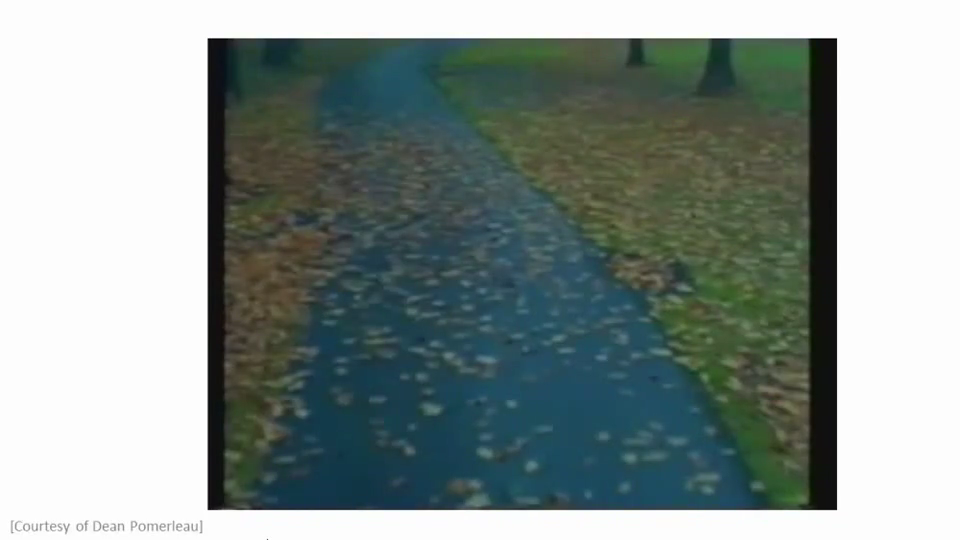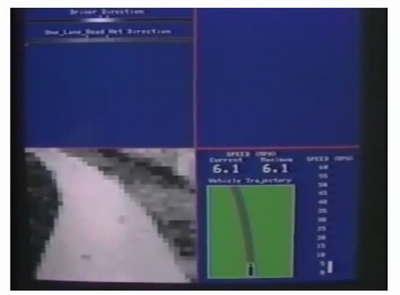# 第5周

## 九、神经网络的学习(Neural Networks: Learning)

### 9.1 代价函数

$K$类分类：$S_L=k, y_i = 1$表示分到第$i$类；$(k>2)$$J\left(\theta \right)=-\frac{1}{m}\left[\sum_\limits{i=1}^{m}{y}^{(i)}\log{h_\theta({x}^{(i)})}+\left(1-{y}^{(i)}\right)log\left(1-h_\theta\left({x}^{(i)}\right)\right)\right]+\frac{\lambda}{2m}\sum_\limits{j=1}^{n}{\theta_j}^{2}$

$J(\Theta) = -\frac{1}{m} \left[ \sum\limits_{i=1}^{m} \sum\limits_{k=1}^{k} {y_k}^{(i)} \log \subk{(h_\Theta(x^{(i)}))} + \left( 1 - y_k^{(i)} \right) \log \left( 1- \subk{\left( h_\Theta \left( x^{(i)} \right) \right)} \right) \right] + \frac{\lambda}{2m} \sum\limits_{l=1}^{L-1} \sum\limits_{i=1}^{s_l} \sum\limits_{j=1}^{s_l+1} \left( \Theta_{ji}^{(l)} \right)^2$

### 9.2 反向传播算法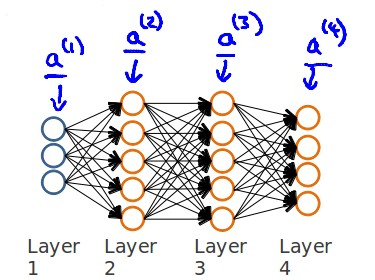$l$ 代表目前所计算的是第几层。

$j$ 代表目前计算层中的激活单元的下标，也将是下一层的第$j$个输入变量的下标。

$i$ 代表下一层中误差单元的下标，是受到权重矩阵中第$i$行影响的下一层中的误差单元的下标。$D_{ij}^{(l)} :=\frac{1}{m}\Delta_{ij}^{(l)}$ ${if}\; j = 0$

Octave 中，如果我们要使用 fminuc这样的优化算法来求解求出权重矩阵，我们需要将矩阵首先展开成为向量，在利用算法求出最优解后再重新转换回矩阵。

​xthetaVec = [Theta1(:) ; Theta2(:) ; Theta3(:)]​...optimization using functions like fminuc...​Theta1 = reshape(thetaVec(1:110, 10, 11);​Theta2 = reshape(thetaVec(111:220, 10, 11);​Theta1 = reshape(thetaVec(221:231, 1, 11);

### 9.3 反向传播算法的直观理解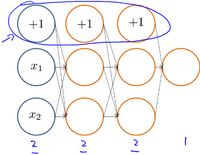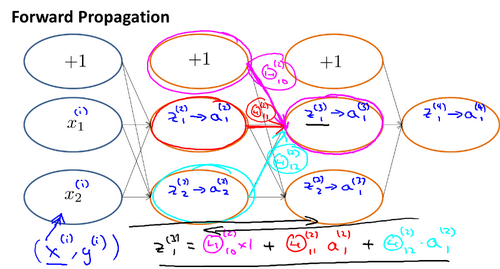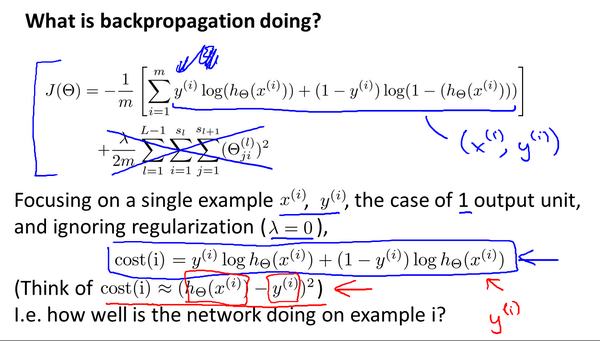### 9.4 实现注意：展开参数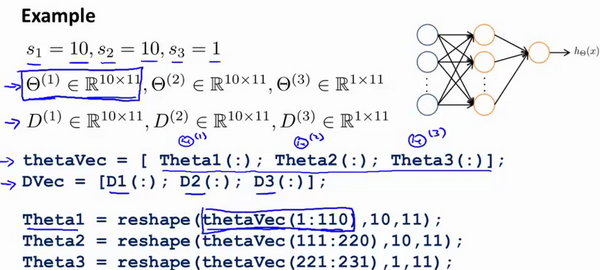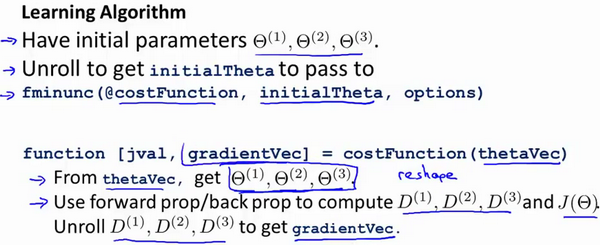### 9.5 梯度检验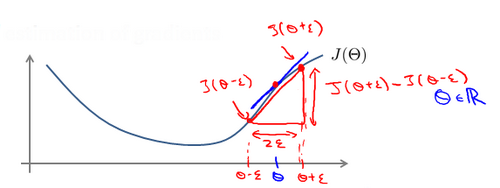Octave 中代码如下：

gradApprox = (J(theta + eps) – J(theta - eps)) / (2*eps)

$\theta$是一个向量时，我们则需要对偏导数进行检验。因为代价函数的偏导数检验只针对一个参数的改变进行检验，下面是一个只针对$\theta_1$进行检验的示例： $\frac{\partial}{\partial\theta_1}=\frac{J\left(\theta_1+\varepsilon_1,\theta_2,\theta_3...\theta_n \right)-J \left( \theta_1-\varepsilon_1,\theta_2,\theta_3...\theta_n \right)}{2\varepsilon}$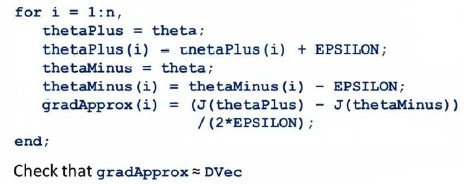### 9.6 随机初始化

Theta1 = rand(10, 11) * (2*eps) – eps

### 9.7 综合起来

1. 参数的随机初始化
2. 利用正向传播方法计算所有的$h_{\theta}(x)$
3. 编写计算代价函数 $J$ 的代码
4. 利用反向传播方法计算所有偏导数
5. 利用数值检验方法检验这些偏导数
6. 使用优化算法来最小化代价函数

### 9.8 自主驾驶ALVINN (Autonomous Land Vehicle In a Neural Network)是一个基于神经网络的智能系统，通过观察人类的驾驶来学习驾驶，ALVINN能够控制NavLab，装在一辆改装版军用悍马，这辆悍马装载了传感器、计算机和驱动器用来进行自动驾驶的导航试验。实现ALVINN功能的第一步，是对它进行训练，也就是训练一个人驾驶汽车。